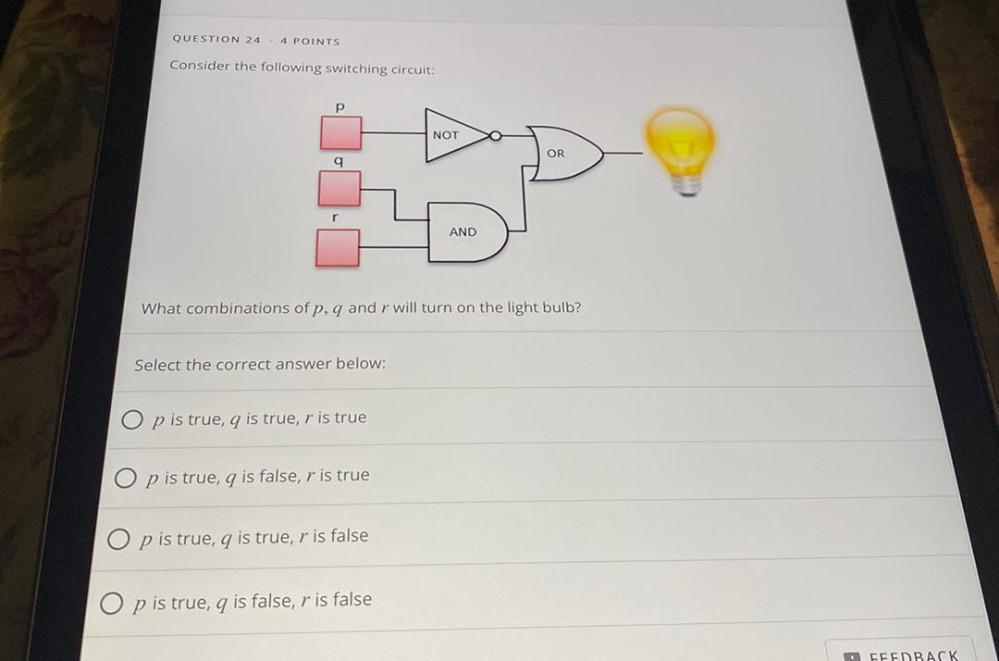Question:

# QUESTION 24 4 POINTS Consider the following switching circuit: p NOT OR q r AND What combinations of p. q and r will turn on theQUESTION 24 4 POINTS Consider the following switching circuit: p NOT OR q r AND What combinations of p. q and r will turn on the light bulb? Select the correct answer below: O p is true, q is true, r is true p is true, q is false, r is true O p is true, 9 is true, r is false O p is true, 9 is false, r is false FEEDBACK